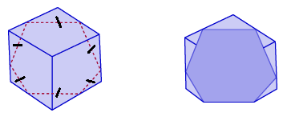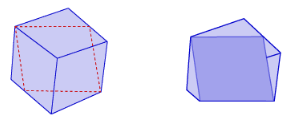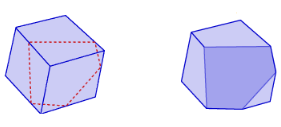# Math in Focus Grade 7 Chapter 8 Lesson 8.5 Answer Key Real-World Problems: Composite Solids

This handy Math in Focus Grade 7 Workbook Answer Key Chapter 8 Lesson 8.5 Real-World Problems: Composite Solids detailed solutions for the textbook questions.

## Math in Focus Grade 7 Course 2 B Chapter 8 Lesson 8.5 Answer Key Real-World Problems: Composite Solids

### Math in Focus Grade 7 Chapter 8 Lesson 8.5 Guided Practice Answer Key

Solve.

Question 1.
A composite solid is made up of a cone and a cylinder. The slant height of the cone is 25 centimeters. The height of the cylinder is 15 centimeters and its radius is 12 centimeters. The height of the solid is 37 centimeters. Use 3.14 as an approximation for π.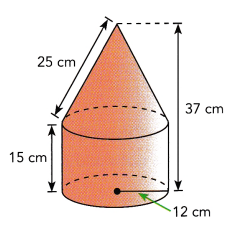a) Find the volume of the composite solid to the nearest cubic centimeter.
Volume of the cylinder:
πr2h =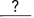Use the formula for volume of a cylinder.
=cm3 Evaluate .

Volume of the cone:
Height of cone ==cm
$$\frac{1}{3}$$ πr2h = $$\frac{1}{3}$$ ∙Use the formula for the volume of a cone.
=cm3 Multiply.
Volume of the composite solid:+=Add the volumes of the cylinder and the cone.cm3 Multiply and round.
The volume of the composite solid is aboutcubic centimeters.
We are given:
l = 25
hcylinder = 15
H = 37
r = 12
Use the formula for the volume of a cylinder:
Vcylinder = πr2hcylinder
= π · 122 · 15
Evaluate:
= 2,160π cm3
The height of the cone:
hcone = H – hcylinder
= 37 – 15
= 22 cm
Use the formula for the volume of a cone:
Vcone = $$\frac{1}{3}$$πr2hcone
= $$\frac{1}{3}$$ · π · 122 · 22
Multiply:
= 1,056π cm3
Find the volume of the composite solid by adding the volumes of the cylinder and the cone:
V = Vcylinder + Vcone
= 2,160π + 1,056π
= 3,216π cm3
Multiply and round:
≈ 10,098 cm3

b) Find the surface area of the composite solid to the nearest square centimeter.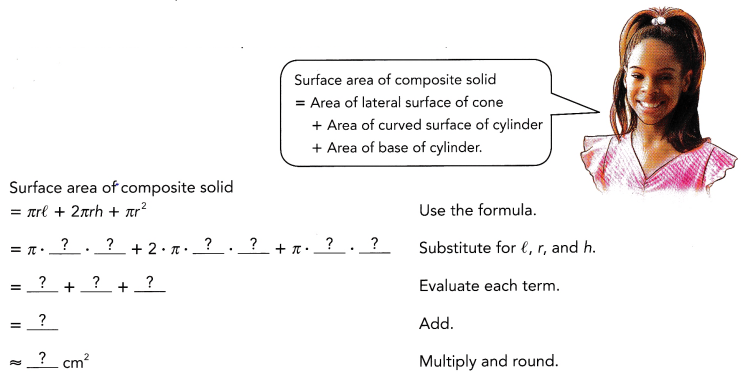The surface area of the composite solid is aboutsquare centimeters.
Answer: The surface area of the composite solid is about  2,525 cm2.
Use the formuLas for the surface areas:
S = πrl + 2πrhcylinder + πr2
Substitute for r, l, hcylinder
= π · 12 · 25 + 2π · 12 · 15 + π · 122
Evaluate each term:
= 300π + 360π + 144π
= 804π
Multiply and round:
≈ 804 · 3.14 ≈ 2,525 cm2

Question 2.
A birdhouse looks like a cube with a square pyramid on top. As shown, the birdhouse has a circular entrance with a diameter of 4 inches. Find the exterior surface area of the birdhouse.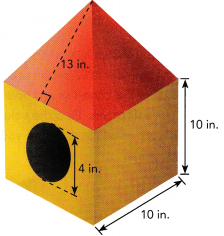Answer: The exterior surface area of the birdhouse is 647.4 in2
Determine the Lateral surface area of the pyramid:
S1 = 4 · $$\frac{1}{2}$$ · 10 · 13 = 260 in2
Determine the radius of the entrance:
r = $$\frac{4}{2}$$ = 2
Determine the lateral surface area of the house:
S2 = 4 · 10 · 10 – π · 22 ≈ 387.4 in2
Compute the exterior surface area of the house:
S = S1 + S2 = 260 + 387.4 = 647.4 in2
= 647.4 in2

Question 3.
The solid shown is a cylinder with a cone-shaped hole. The diameter of the cylinder is 22 centimeters. Its height is 15 centimeters. The radius of the cone-shaped hole is 7 centimeters and the height is 10 centimeters. Find the volume of the solid. Use 3.14 as an approximation for π. Round your answer to the nearest cubic centimeter.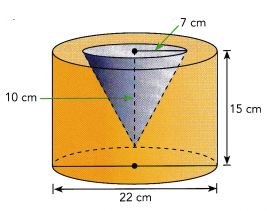Answer: The volume of the solid is  5. 187 cm3
Determine the radius R of the cylinder:
R = $$\frac{22}{2}$$ = 11 cm
Determine the volume of the cylinder
Vcylinder = πR2h = π · 112 ·  15 = 1, 815π cm2
Determine the volume of the cone:
Vcone = $$\frac{1}{3}$$πR2h = $$\frac{1}{3}$$ · π · 72 · 10 ≈ 163π cm3
Determine the volume of the solid:
V = Vcylinder – Vcone
= 1,815π – 163π
= 1,652π
≈ 3.14 · 1,652
≈ 5. 187 cm3

### Math in Focus Course 2B Practice 8.5 Answer Key

For this practice, you may use a calculator and use 3.14 as an approximation for π. Round your answers to the nearest tenth when you can.

Solve.

Question 1.
Jack has a cylindrical block that has a radius of 0.6 cm and is 22 centimeters long. He puts together 8 such blocks to form the composite solid shown. What is the volume of the composite solid?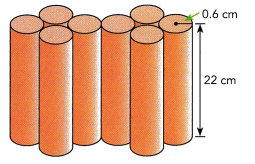Answer: The volume of the composite solid is 199 cm3
We are given the cylinder:
r = 0.6
h = 22
Determine the volume of one cylinder:
Vcylinder = πr2h ≈ 3.14 · 0.62 · 22
≈ 24.87 cm3
Determine the volume of the composite solid:
Vsolid = 8Vcylinder = 8 · 24.87 ≈ 199 cm3

Question 2.
The trophy for a basketball tournament is made up of a miniature basketball attached to a rectangular prism. The radius of the basketball is 5 centimeters. The prism measures 8 centimeters by 5 centimeters by 15 centimeters. What is the volume of the trophy to the nearest cubic centimeter?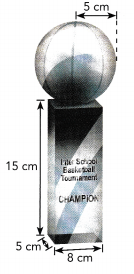Answer: The volume of the trophy is 1,123 cm3
We are given:
r = 5
l = 8
w = 5
h = 15
Determine the volume of the sphere:
Vsphere = $$\frac{4}{3}$$πr3
≈ $$\frac{4}{3}$$ · 3.14 · 53
≈ 523 cm3
Determine the volume of the prism:
Vprism = lwh = 8 · 5 · 15 = 600 cm3
Determine the volume of the trophy:
Vtrophy = Vsphere + Vprism
= 523 + 600
= 1,123 cm3

Question 3.
a) What is the volume of the necklace?
Answer: The volume of the necklace is 107, 178.7 mm3
We are given the sphere:
r = 8
Determine the volume of a spherical bead:
Vbead = $$\frac{4}{3}$$πr3
≈ $$\frac{4}{3}$$ · 3.14 · 83
≈ 2,143.573
Determine the volume of the necklace:
≈ 50 – 2. 143.573 ≈ 107, 178.7 mm3

b) What is the surface area of the necklace?
Answer: The surface area of the necklace is 40,192 mm2
Determine the surface area of a spherical bead:
Sbead = 4πr2 ≈ 4 · 3.14 · 82
≈ 803.84 mm2
Determine the surface area of the necklace:
= 50 · 803.84
≈ 40,192 mm2

Question 4.
A crystal trophy is made up of a rectangular pyramid whose base is attached to the top of a rectangular prism. The base of the pyramid and the top of the prism are each 4 inches long and 3 inches wide. The height of the pyramid is 2.5 inches and the height of the prism is 9.5 inches. What is the volume of the crystal trophy?
Answer: The volume of the crystal trophy is 124 in3
We are given:
l = 4
w = 3
hpyramid = 2.5
hprism = 9.5
Determine the volume of the pyramid:
Vpyramid =$$\frac{1}{3}$$ · lwhpyramid
= $$\frac{1}{3}$$ · 4 · 3 · 2.5
= 10 in3
Determine the volume of the prism:
Vprism = lwhprism = 4 · 3 · 9.5
= 114 in3
Determine the volume of the trophy:
Vtrophy = Vpyramid + Vprism
= 10 + 114
= 124 in3

Question 5.
At a food stand, you can buy a paper cone filled with slush made of frozen juice. The slush forms a hemisphere on top of the cone, as shown. What is the volume of the cone of slush?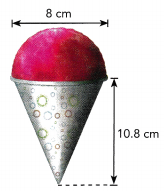Answer: The volume of the cone of slush is 314.9 cm3
We are given:
d = 8
h = 10.8
r = $$\frac{d}{2}$$ = $$\frac{8}{2}$$ = 4
Determine the volume of the hemisphere:
Vhemisphere = $$\frac{1}{2}$$ · $$\frac{4}{3}$$ πr3
≈ $$\frac{2}{3}$$ · 3.14 · 43
≈ 134 cm3
Determine the volume of the cone:
Vcone = $$\frac{1}{3}$$ πr2h
≈ $$\frac{1}{3}$$ · 3.14 · 42 · 10.8
≈ 180.9 cm3
Determine the volume of the cone of slush:
V = Vhemisphere + Vcone
= 134 + 180.9
= 314.9 cm3

Question 6.
A wooden paper towel holder is composed of two cylinders. The diameter of the base is 12 .centimeters and its height is 2 centimeters. The combined height of the two cylinders is 30 centimeters. What is the volume of the paper towel holder?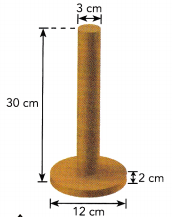Answer: The volume of the paper towel holder is 438 cm3
We are given:
d1 = 3
h1 = 30
d2 = 12
h2 = 2
Determine the radius of the upper cylinder:
r1 = $$\frac{d_{1}}{2}$$ = $$\frac{3}{2}$$ = 1.5
Determine the volume of the upper cylinder:
V1 = πr12h1 = π · 1 52 · 30 = 67.5π cm3
Determine the radius of the lower cylinder:
r2 = $$\frac{d_{2}}{2}$$ = $$\frac{12}{2}$$ = 6
Determine the volume of the lower cylinder:
V2 = πr22h2 = π · 62 · 2 = 72π cm3
Determine the volume of the paper tower holder:
V = V1 + V2 = 67.5π + 72π
≈ 139.5π
≈ 139.5 · 3.14
≈ 438 cm3

Question 7.
The edge of the base of a square pyramid is 11 inches. The pyramid has a height of 14 inches. What is the volume of the composite solid formed when two such pyramids are joined at the base, as shown in the diagram?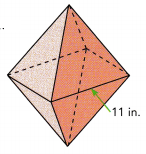Answer: The volume of the composite solid is  1,129.3 in3
We are given:
L = 11
h = 14
Determine the volume of one pyramid:
V1 = V2 = $$\frac{1}{3}$$l2h = $$\frac{1}{3}$$ · 112 · 14 ≈ 564.67 in3
Determine the volume of the composite solid:
V = V1 + V2 = 2V1 = 2 · 564.67
≈ 1,129.3 in3

Question 8.
A clock in the shape of an hour glass is made up of two identical cones connected at their vertices. The radius of each cone is 7 centimeters. The combined height of the two cones is 11.8 centimeters. What is the volume of the clock?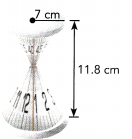Answer: The volume of the clock is 605.2 cm3
We are given:
r = 7
H = 11.8
Determine the height of each cone:
h = $$\frac{H}{2}$$ = $$\frac{11.8}{2}$$ = 5.9
Determine the volume of one cone:
V1 = V2 = $$\frac{1}{3}$$πr2h =  $$\frac{1}{3}$$ · π · 72 · 59
≈ 96.3677π
Determine the volume of the clock:
V = V1 + V2 = 2V1 = 2 · 96.367π
≈ 192.734 · 3.14
≈ 605.2 cm3

Question 9.
Jason made a long pole by joining three different lengths of cylindrical poles together. Each pole has the same diameter of 18 cm. The diagram below is not drawn to scale.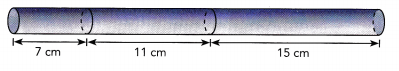a) What is the volume of the long pole?
Answer: The volume of the long pole is 8,393.2 cm3
We are given:
d = 18
h1 = 7
h2 = 11
h3 = 15
r = $$\frac{d}{2}$$ = $$\frac{18}{2}$$ = 9
Determine the volume of the tong pole:
V = V1 + V2 + V3
= πr2h1 + πr2h2 + πr2 h3
= πr2(h1 + h2 + h3)
≈ 3.14 · 92 · (7 + 11 + 15)
≈ 8,393.2 cm3

b) What is the surface area of the long pole?
Answer: The surface area of the long pole is  2,373.8 cm2
Determine the surface area of the long pole
= 2πr2 + 2πr(h1 + h2 + h3)
= 2πr(r + h1 + h2 + h3)
≈ 2 · 3.14 · 9(9 + 7 + 11 + 15)
≈ 2,373.8 cm2

Brain @ Work

How can you make the following cross-sections by slicing a cube? Use a computer drawing program or pencil and paper to show your answers for a) and b).
a) An isosceles triangle
We take a point on one edge, then take two congruent segments from one of the vertices on the same edge on the other two edges
We draw the cross-section in the cube in the shape of an isosceles triangle: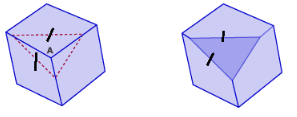b) A regular hexagon